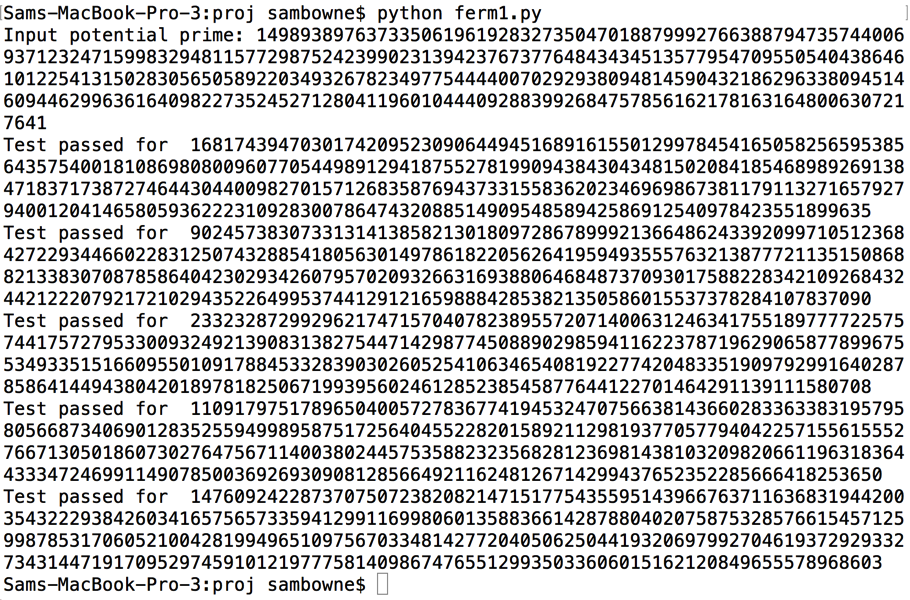# Finding Large Primes

What you need:

## Purpose

To implement the Fermat primality test and find large primes.

## Background

Fermat's little theorem states that if p is prime and a is not divisible by p, then:So testing that condition for several random values of a can be used as evidence that p is probably prime.

This site explains the Fermat primality test and its flaws in more detail:

## Simple Python Implementation

```from random import randint p = int(raw_input("Input potential prime: ")) for i in range(5): a = randint(2,p-2) if pow(a, p-1, p) == 1: print "Test passed for ", a else: print "Test failed for ", a ```

## Demonstration

Try these values: 11, 13, 100, 121.

As shown below, 11 and 13 pass all the tests because they are prime, but 100 and 121 fail because they aren't.## Testing Large Numbers

Test this number, which is 1024 bits long, and is prime:

149893897637335061961928327350470188799927663887947357440069371232471599832948115772987524239902313942376737764843434513577954709550540438646101225413150283056505892203493267823497754444007029293809481459043218629633809451460944629963616409822735245271280411960104440928839926847578561621781631648006307217641

It works, because Python really can handle any size of integer, as shown below.## Challenge 1: Find the Next Prime (10 pts. extra credit)

This number is not prime:

10**300

Find the first number above it which is prime.

Use the form below to put your name on the WINNERS PAGE.

 Your Name (without spaces): Answer, as one long integer, like 11111111111111111111111111111111 :

## Challenge 2: Companion Primes (10 pts. extra credit)

11 and 13 are both primes, only differing by two. Such primes are called companion primes.

Find the first set of companion primes above

10**100

Use the form below to put your name on the WINNERS PAGE.

 Your Name (without spaces): The lower of the two primes, as one long integer, like 11111111111111111111111111111111 :

Posted 11-1-17 by Sam Bowne
Added to Crypto Hero 4-16-18 6:26 am